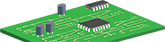# Arduino with PIR

Hi friends! I am back ones again to share with you my simple but useful project and step by step descriptive video to show you, how you can auto turn on the room Light when some come inside the room. It’s only work when someone moving in the room and after few second light will turn off automatically. To detect the motion I have used PIR Motion Sensor and program the arduino board to read the sensor signal and turn on the light for few second.

List of components to make this prototype:

Arduino Uno

PIR motion sensor

LED

Jumper wire

PIR motion sensor with Arduino video tutorial

### Arduino code for PIR Motion Sensor prototype

`intcalibrationTime = 30;`

`//the time when the sensor outputs a low impulse`

`longunsigned intlowIn;`

`//the amount of milliseconds the sensor has to be low`

`//before we assume all motion has stopped`

`longunsigned intpause = 5000;`

`boolean lockLow = true;`

`boolean takeLowTime;`

`intpirPin = 12; //the digital pin connected to the PIR sensor's output`

`intledPin = 13;`

`////`

`//SETUP`

`voidsetup(){`

`Serial.begin(9600);`

`pinMode(pirPin, INPUT);`

`pinMode(ledPin, OUTPUT);`

`digitalWrite(pirPin, LOW);`

`//give the sensor some time to calibrate`

`Serial.print("calibrating sensor ");`

`for(inti = 0; i < calibrationTime; i++){`

`Serial.print(".");`

`delay(1000);`

`}`

`Serial.println(" done");`

`Serial.println("SENSOR ACTIVE");`

`delay(50);`

`}`

`//LOOP`

`voidloop(){`

`if(digitalRead(pirPin) == HIGH){`

`digitalWrite(ledPin, HIGH); //the led visualizes the sensors output pin state`

`if(lockLow){`

`lockLow = false;`

`Serial.println("---");`

`Serial.print("motion detected at ");`

`Serial.print(millis()/1000);`

`Serial.println(" sec");`

`delay(50);`

`}`

`takeLowTime = true;`

`}`

`if(digitalRead(pirPin) == LOW){`

`digitalWrite(ledPin, LOW); //the led visualizes the sensors output pin state`

`if(takeLowTime){`

`lowIn = millis(); //save the time of the transition from high to LOW`

`takeLowTime = false;`

`}`

`//if the sensor is low for more than the given pause,`

`//we assume that no more motion is going to happen`

`if(!lockLow && millis() - lowIn > pause){`

`//makes sure this block of code is only executed again after`

`//a new motion sequence has been detected`

`lockLow = true;`

`Serial.print("motion ended at "); //output`

`Serial.print((millis() - pause)/1000);`

`Serial.println(" sec");`

`delay(50);`

`}`

`}`

`}`

Thanks

pcbway

Sep 28,2019
646 viewsReport item
• Likes(0)
You can only upload 1 files in total. Each file cannot exceed 2MB. Supports JPG, JPEG, GIF, PNG, BMP
View More

• ### Universal motor driver and control board version 2x
mm
Earn a \$5 Welcome Bonus